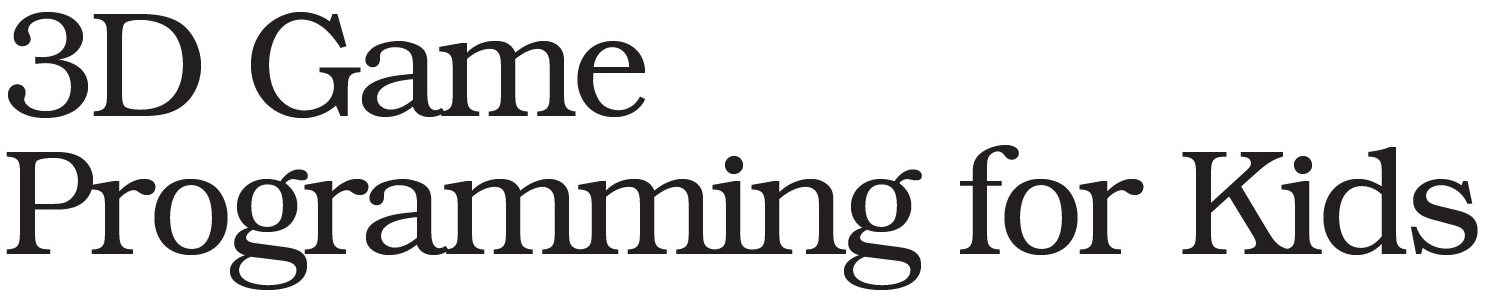# Chapter V Problems of Flight Control

I am a Chinese, and the following content comes from Baidu Translator:
My editor has a problem like the one above. The result area is my code.
When I went to the flight control phase of Chapter 5, it became like this. I can guarantee that I have checked the console
Here is my code:

// The "scene" is where stuff in our game will happen: var scene = new THREE.Scene(); var flat = {flatShading: true}; var light = new THREE.AmbientLight('white', 0.8); scene.add(light); // The "camera" is what sees the stuff: var aspectRatio = window.innerWidth / window.innerHeight; var camera = new THREE.PerspectiveCamera(75, aspectRatio, 1, 10000); camera.position.z = 500; scene.add(camera); // The "renderer" draws what the camera sees onto the screen: var renderer = new THREE.WebGLRenderer({antialias: true}); renderer.setSize(window.innerWidth, window.innerHeight); document.body.appendChild(renderer.domElement); // ******** START CODING ON THE NEXT LINE ******** function r(max){ if (max) return max*Math.random(); return Math.random(); } function rc(){ return new THREE.Color(r() , r() , r()); } function mP(){ var size = r(50); var x = r(1000)-500; var y = r(1000)-500; var z = r(1000)-1000; var surface = rc(); var shape = new THREE.SphereGeometry(size); var cover = new THREE.MeshBasicMaterial({color: surface}); var planet = new THREE.Mesh(shape,cover); planet.position.set(x,y,z); scene.add(planet); } for (var i=0;i<100;i++){ mP(); } var controls = new THREE.FlyControls(camera); controls.movementSpeed = 100; controls.rollSpeed = 0.5; controls.dragToLook = true; controls.autoForward = false; var clock = new THREE.Clock(); function animate(){ var delta = clock.getDelta; controls.update(delta); renderer.render(scene, camera); requestAnimationFrame(animate); } animate(); thank you

You have typed all of your code correctly – except for one line.

Inside the `animate()` function, change the following line:

``````    var delta = clock.getDelta;
``````

Change it to:

``````    var delta = clock.getDelta();
``````

The entire code should then be:

``````<body></body>
<script src="/three.js"></script>
<script src="/controls/FlyControls.js"></script>
<script>
// The "scene" is where stuff in our game will happen:
var scene = new THREE.Scene();
var light = new THREE.AmbientLight('white', 0.8);

// The "camera" is what sees the stuff:
var aspectRatio = window.innerWidth / window.innerHeight;
var camera = new THREE.PerspectiveCamera(75, aspectRatio, 1, 10000);

// The "renderer" draws what the camera sees onto the screen:
var renderer = new THREE.WebGLRenderer({antialias: true});
renderer.setSize(window.innerWidth, window.innerHeight);
document.body.appendChild(renderer.domElement);

// ******** START CODING ON THE NEXT LINE ********
function r(max){
if (max) return max*Math.random();
return Math.random();
}
function rc(){
return new THREE.Color(r() , r() , r());
}

function mP(){
var size = r(50);
var x = r(1000)-500;
var y = r(1000)-500;
var z = r(1000)-1000;
var surface = rc();
var shape = new THREE.SphereGeometry(size);
var cover = new THREE.MeshBasicMaterial({color: surface});
var planet = new THREE.Mesh(shape,cover);
planet.position.set(x,y,z);
}

for (var i=0;i<100;i++){ mP(); }

var controls = new THREE.FlyControls(camera);
controls.movementSpeed = 100;
controls.rollSpeed = 0.5;
controls.dragToLook = true;
controls.autoForward = false;

var clock = new THREE.Clock();
function animate(){
var delta = clock.getDelta();
controls.update(delta);
renderer.render(scene, camera);
requestAnimationFrame(animate);
}
animate();
</script>
``````

Let me know if you have any other questions.

-Chris

Thank you, but I have another question.
This problem is discussed in Chapter 11
I found that characters can’t jump, but they can get extra points.
Console display:
at aJU (code.html:319:47)
at aJ (code.html:313:20)
at Jump (code.html:292:5)
at HTMLDocument.sendKeyDown (code.html:338:26)

I’ll need to see your code in order to help you. Either use the share option from the menu in 3DE or copy and paste your code directly into your reply. The share option is less error prone. If you prefer copy & paste, be sure to paste it between 3 backtick lines:

``````Explain how your code is misbehaving...

```